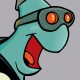# costmap_2d_ros always filled with lethal obstacles [closed]I have made a node that keeps a local costmap_2d_ros object but currently it seems that the costmap is filled with all lethal obstacles (value 254) and I am unable to clear it. I based my code off of the code in the move_base package. I have pasted the relevant code below. In summary, I use the methods getCostmapCopy and getCharMap and print out the values in the char array. All the values are 254 and I then try using resetMapOutsideWindow with the window size being 0,0. The values are still all 254. Any advice would be greatly appreciated and if you need any more information I'd be happy to supply it.

namespace matrix_encoder {
class MatrixEncoder {
public:
MatrixEncoder(std::string name, tf::TransformListener& tf);
//~MatrixEncoder();
private:
tf::TransformListener& tf_;
costmap_2d::Costmap2DROS* encoder_costmap_ros;
costmap_2d::Costmap2D costmap; // The underlying costmap to update
const unsigned char* charArray;  // pointer to the underlying unsigned char array used as the costmap
};
};

namespace matrix_encoder {

MatrixEncoder::MatrixEncoder(std::string name, tf::TransformListener& tf) :
tf_(tf), encoder_costmap_ros(NULL), charArray(NULL) {

ros::NodeHandle nh;

encoder_costmap_ros = new costmap_2d::Costmap2DROS("encoder_costmap", tf_);
encoder_costmap_ros->getCostmapCopy(costmap);
charArray = costmap.getCharMap();
int index = 0;
for (int j = 0; j < numXcells; j++) {
for(int i = 0; i < numYcells; i++) {
ROS_INFO("%d", charArray[index++]);
}
}

index = 0;
encoder_costmap_ros->resetMapOutsideWindow(0,0);
encoder_costmap_ros->getCostmapCopy(costmap);
charArray = costmap.getCharMap();
for (int j = 0; j < numXcells; j++) {
for(int i = 0; i < numYcells; i++) {
ROS_INFO("%d", charArray[index++]);
}
}
}

int main(int argc, char **argv)
{
ros::init(argc, argv, "encoder");

tf::TransformListener tf(ros::Duration(10));
matrix_encoder::MatrixEncoder matrix_encoder("matrix_encoder", tf);

ros::NodeHandle n;

ros::spin();

return 0;
}

edit retag reopen merge delete